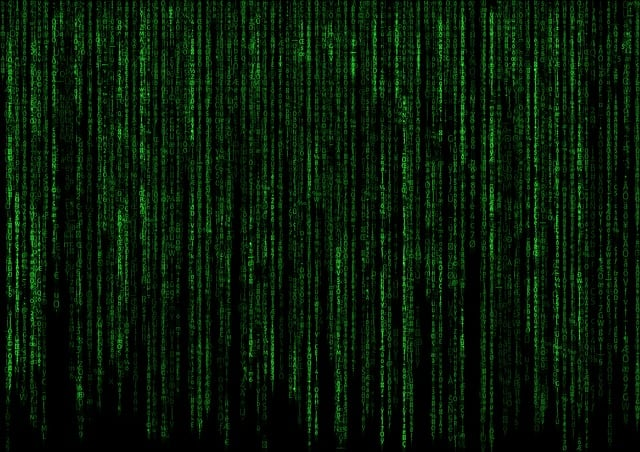# Understanding Python 3 data types: string, int, float and boolean

Introduction to Python 3&#39;s primitive data types: int, float, boolean and string

In programming languages, a data structure is a way of organizing and structuring pieces of data, and a data type is just a piece of data. Data structures can be divided into two categories: primitive and non-primitive data structures. Primitive data structures are the simplest forms of representing data hence the name primitive, where the non-primitive structures are designed to organize and manage sets of primitive data. For more information about non-primitive data structures, see this article: Data structures in Python.

## Primitive data types

Python has four primitive data types:

• Integer

• Float

• String

• Boolean

In the next section, we will go through the various primitive data types in Python.

### Integer

Just as in mathematics (where it is referred to as a signed integer) an integer is a whole number that could hold a zero, positive or negative value. This is how you would create various integers in Python:

# positive number
number = 25

# negative number
negative_number = -23

zero = 0


In Python 3 there are no different integer types as in Python 2.7, which has int and long int. In Python 3 there is only one integer type, which can store very large numbers. For example:

number = 100000000000000000000000
print(type(number))

# output in Python 3 will be
<type 'int'>


Python has built-in methods for converting between integers and floats. You can convert any decimal number, octal, hexadecimal or string to an int by using the int() function. For example:

decimal_number = 23.5print(int(decimal_number))# prints 23
year = "1999"print(int(year))# prints 1999
# for hexadecimal also provide base - Base of the number in x.print("int is:", int('0xA', 16))
# prints 'int is: 10'


### Float

Float represents real numbers, a data type that is used to define floating decimal points. These are some examples of float numbers in Python:

decimal_number = 25.33
decimal_number_two = 45.2424


To check if something is a float we can use the isinstance() function, e.g:

isinstance(4.5, float)# returns True


As a general rule integers don't have a decimal point, whereas floats do, so 55 and 55.0 have the same value but they are different types. Using the float() function each string or integer could be changed to a float, e.g:

number = 5print(float(number))# returns 5.0


The round() function returns the provided number rounded to a number of digits from the decimal point, e.g:

print("Number is rounded to two digits: (50.13456, 2) : ", round(50.13456, 2))# prints Number is rounded to two digits: (50.13456, 2) :  50.13
print("Number is rounded to four digits: (15.456787, 4) : ", round(15.456787, 4))# prints Number is rounded to four digits: (15.456787, 4) :  15.4568


### String

String represents a sequence of characters (text) inside double or single quotes. In Python, strings are immutable so once it's declared the value can't be changed, instead a new object is created e.g:

first_string = "Hello"second_string = first_stringprint(first_string)# prints 'Hello'
print(second_string)# prints 'Hello'
# let's change first_stringfirst_string = "I have changed"print(second_string)# prints 'Hello'


From the previous example, we can see the difference between mutating an object and changing a reference. By assigning a value "I have changed" to first_string it only changes the reference, while the String object it originally referred to remains unchanged.

With Python 3 all strings are now Unicode strings, e.g:

name = 'Zoügel'print(name)# prints 'Zoügel'


Also, the string class has a lot of useful methods for string manipulation, e.g capitalize():

first_name = 'john'print(first_name.capitalize())# prints 'John'


Here's an example of the string formatting method: format() or f-strings_:_

"The sum of 5 + 5 is {0}".format(5+5)# prints 'The sum of 5 + 5 is 10'
name = 'Zoran'age = 28f'My name is {name} and I'm {age} years old.'# returns My name is Zoran and I', 28 years old.


You can also check if string characters are lowercase using islower(), or uppercase using isupper():

address = "STREET 123"print(address.islower())#returns Falseprint(address.isupper())#returns True


There are plenty of useful methods to check different things like: if the string startswith() or endswith() some word, or check if a string isdigit() or isnumeric() . You can refer to the Python 3 documentation for a full list of string methods.

### Boolean

Booleans are used to represent truth values with two constant objects True and False. The  built-in function for converting an object to Boolean is bool(), e.g:

num = 1print(bool(num))#returns True since Boolean in numeric can be present as 0 or 1


### Conclusion

Python supports type inferencing which is a process for automatic detection of the type of the data, so the keywords that are used for creating data types in most of the programming languages e.g (int, float, boolean) are not required in Python. For more information about data types and their available methods, you can check official documentation.Great post